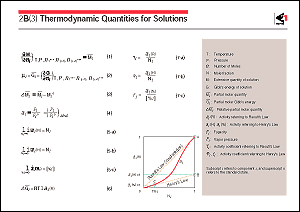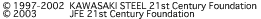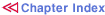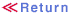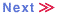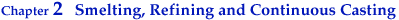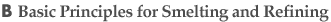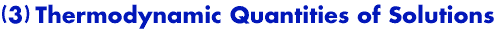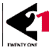Various phases such as molten iron, slag, and gas are all involved in the smelting and refining of iron and steel. Although some of these phases may consist of a single substance or compound, they are in many cases a homogeneous phase composed of multiple components, namely a solution. The extensive quantities such as the free energy, enthalpy, and entropy of a solution undergo changes depending on the quantity and composition of the solution, as well as on its temperature and pressure. However, owing to interaction among the atoms of the solution components, the way in which change actually occurs is not as simple as when elements or compounds are mechanically mixed. Therefore, the partial molar quantity defined in Eq.1 is used to represent various thermodynamic relationships for the extensive quantities of the solution. The suffixes for the partial differential coefficient in Eq.1 show that they are invariable.i can be regarded as the amount of change in the quantity M when only component i is added in an extremely small quantity at a constant temperature and constant pressure, without changing the quantities of the other components. This is defined as the partial molar quantity of component i. Among other partial molar quantities, the partial molar Gibb's energy is important in discussing the activity, etc. of a solution. It is called the chemical potential and is expressed by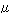as shown in Eq. 2. The difference between the partial molar quantity of a component of a solution and the quantity for the pure state of the component is referred to as the relative partial molar quantity of that component, and is expressed as Eq. 3. Equilibrium of the chemical reactions related to a solution is governed by the activities of these reaction components. Therefore, knowledge of the activities of these components is necessary when considering the equilibria of reactions in smelting and refining and the reactivity of solution components. The activity of a solution component is defined in Eq. 4 as the ratio of the fugacity in the solution component to the fugacity in the standard state of that component (i.e., the ratio of the vapor pressures of the component when its vapor pressure is low). The reference and standard state for activity can be arbitrarily selected. In general, the reference and standard state are adopted as shown in Eqs. 5-a, 5-b and 5-c for the smelting and refining of iron and steel. The figure shows the relationship between the activity and concentration of a solution component, A, B and C representing the standard state of activities in accordance with the respective reference states of Eqs. 5-a, 5-b and 5-c. The relationship in Eq. 6 holds between the relative partial molar Gibb's energy and the activity of an actual solution component. The activity of a component in actual solution deviates from Raoult's law or Henry's law, and hence the activity coefficients defined by Eqs. 7-a, 7-b and 7-c are used to indicate the degree of the deviation. For considering the activity and activity coefficient of the components in a multi-component solution, more detailed knowledge of the thermodynamics, such as interaction coefficients and interaction parameters, is required.